|

# 神奇的空间扩容术,让你的房间不再压抑外,还能再多一间房!

空间扩容是一个没有国界，没有户型大小之分的话题。

要说把扩容做到极致的，应该是日本了，即使在酒店里，都处处看到妥善的空间利用。

先把日本的平价酒店和胶囊旅馆拉出来遛遛，啊不，说说。

胶囊旅馆▲为了保持环境清洁，入门后首先要把鞋子放在大厅大鞋柜中。房君突然想起曾经住过的青旅多人间，因为是公共场合很多人根本不讲究卫生，带着外面的泥土就回来了，下雨下雪的时候更是变态，想把背包放在地上都怕弄脏了。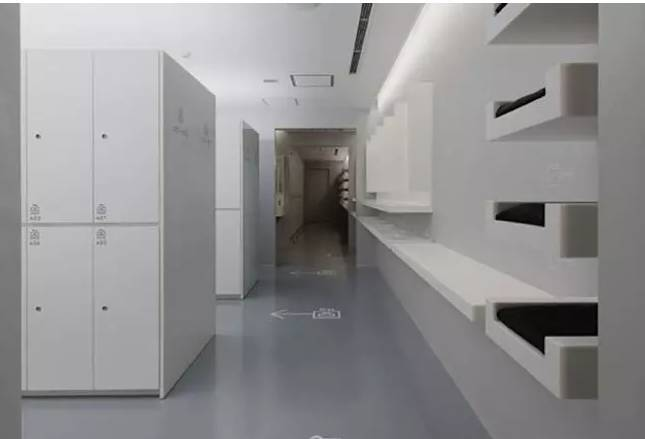▲行李也有专门的储物柜，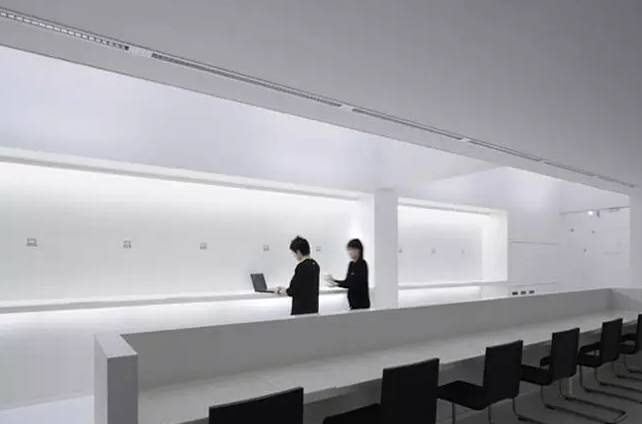▲楼下的休息区由网络和可以租借的电脑▲睡眠区就是一个个的太空舱了，地上的箭头指明了房间号，昏黄的灯光非常有助于睡眠，每个人都是独立的空间，避免了群居毫无隐私的尴尬。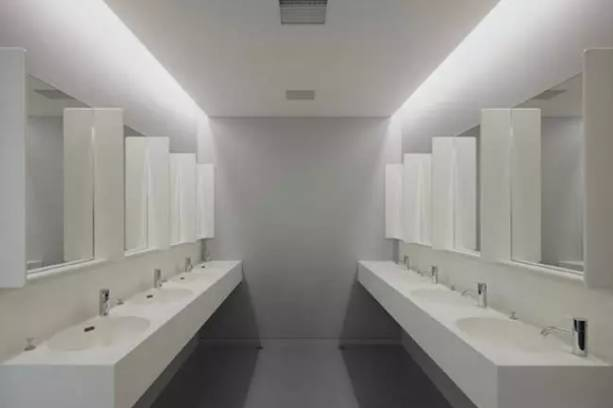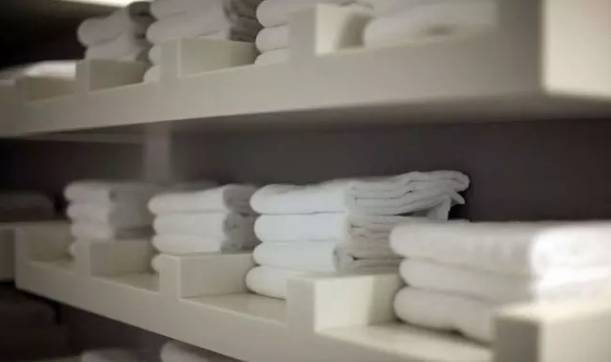▲毛巾、拖鞋等洗漱用品都干净整齐地摆放在洗漱区，洗护用品和瓶装水也都准备的很全。

平价旅馆

日本平价旅馆的配置和国内的差不多，床、床头柜、矮斗柜、梳妆台、椅子，但是有的国内旅馆还配有电视柜。

但是大家看一下，这个日本的旅馆，梳妆台和电视柜融为一体，直接把电视放在梳妆台上，镜子上墙，还能兼办公桌。说胶囊旅馆和平价旅馆呢，是想举个栗子说一下房间功能分区、色调统一、还有家具多功能性，对于家居的重要性。

空间的扩容改造，能解决太多了问题了。

客厅

▽

客餐厨一体化是最能从视觉上增大房间面积的设计了，但是同时又要注意用岛台、屏风、储物柜、地台、地毯等软隔断，来做功能分区。

同时可以适当在墙面上增加搁板来做一个书柜，花草柜，除了收纳外，还是很温馨的装饰。

如果空间实在有限的话，可以用不同的地面材质来划分，这时候小花砖就能派上用场啦。厨房

▽

现在越来越多的人家选择敞开式厨房，甚至很多案例中，一百三四十平的房子也会把客厅和厨房打通，光线好得像巨大的别墅。

而因为厨房敞开，装修就必须与整个空间协调，再不不能随随便便选择一些难看的橱柜，无形中逼着屋主提高自己的审美，装修的时候认认真真做功课。厨房的扩容术更应该讲究。

要有窗户，有阳光射进来的地方才不会有压抑的感觉。

整体色彩要一致、浅色、单调，本来厨房就是面积小东西多，如果颜色再花哨起来，看着就更杂乱了。

多使用搁板、吊柜、挂篮，能上墙的东西就上墙，这些厨房神器都是收纳和装饰的好帮手，当然前提是你的器具一定要颜值高！高！高！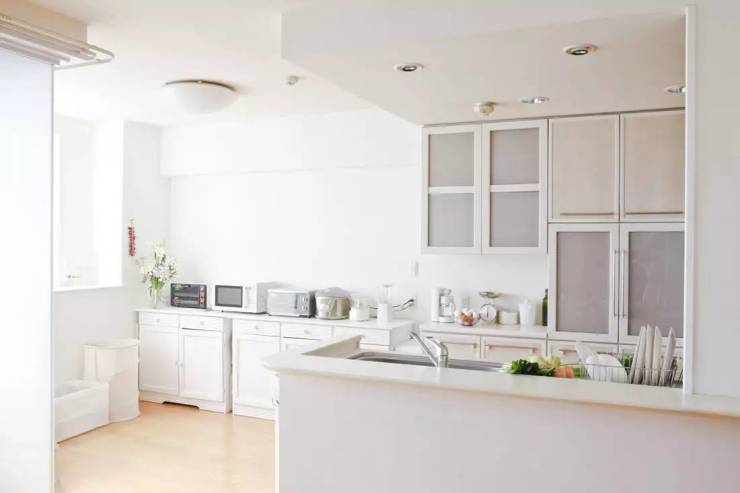榻榻米

▽

房君曾经推送过一篇关于榻榻米的文章，《这才是家居界的百变全能王，让你的小房子越住越大！》里面从头到尾分析了榻榻米除了不是很好打扫外，是个居家神器。

榻榻米真的是好东西，铺上被褥就是床，打开床板是储物柜，摆上小桌子和垫子就是喝茶聊天的休闲区，榻榻米旁边的墙还可以打上搁板做成书架，放假的时候就躺在榻榻米上，喝喝茶，看看书，岂不快哉。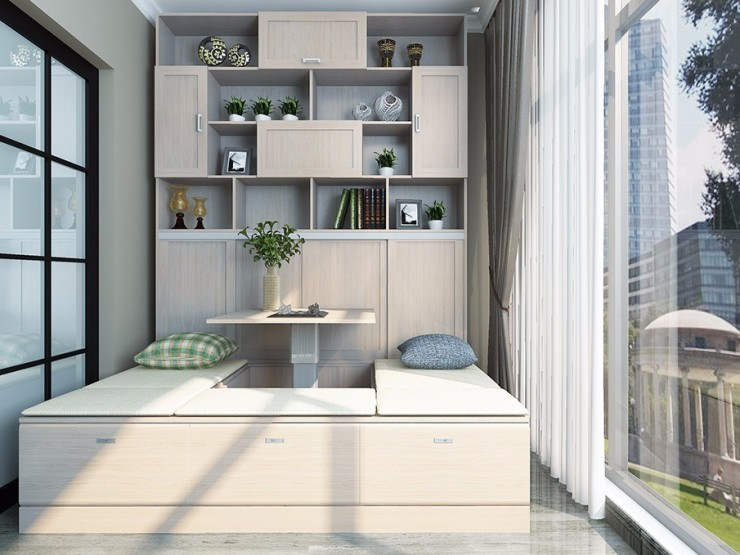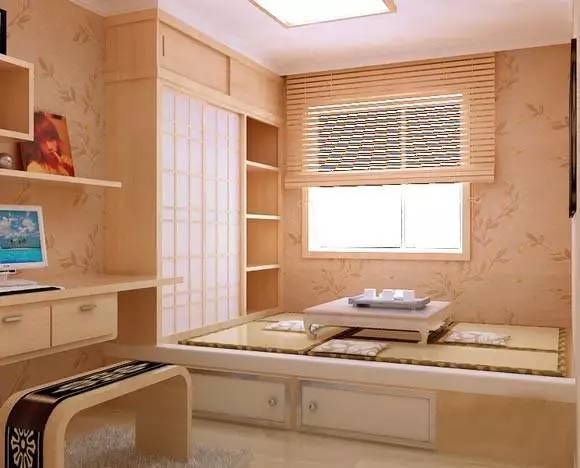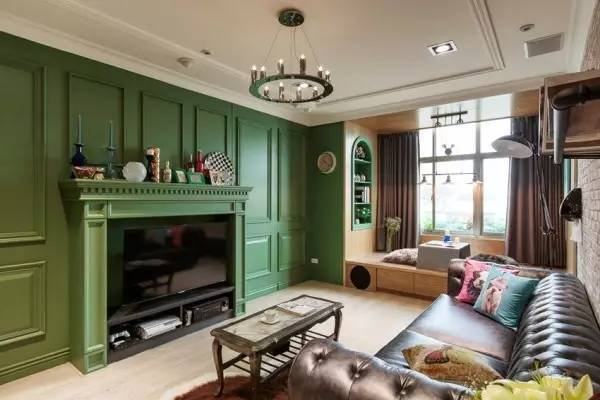轻盈感

▽

营造有轻盈感的家居环境，最重要的事情就是让空间变得通透起来，空间通透了，视觉也就通透了。

尤其是那些面积不够大、光线也不够透亮的空间，更需要那些轻盈、纤细、充满呼吸感的元素来调节了。

文字都是多余的，直接上图体验。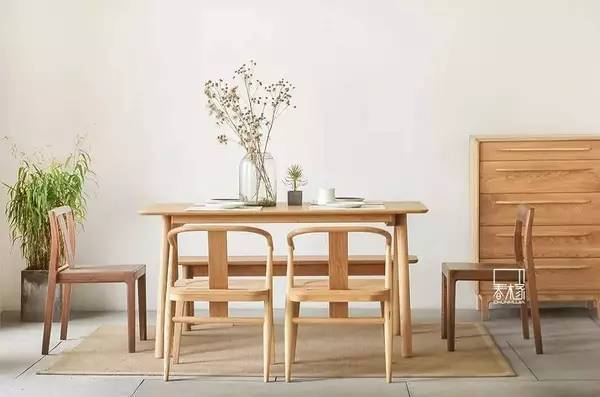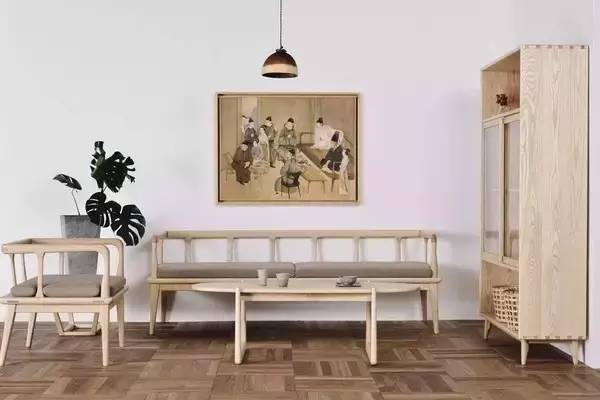留白

▽

留白是中式家装中，很能提升逼格的元素，同时视觉上看起来也很舒适，没有一点局促拥挤的感觉。

房间适当的留白，也是一种很好的选择。矮橱柜

▽

十只柯基在一个空间里，和十只哈士奇在一个空间里，是不是还是柯基看起来更省地方？

家具也是一个道理，一个巨大的书柜和一个矮柜比起来，还是矮柜子更节省空间。

同时矮柜还能起到隔断的作用，隔而不断，视野通透。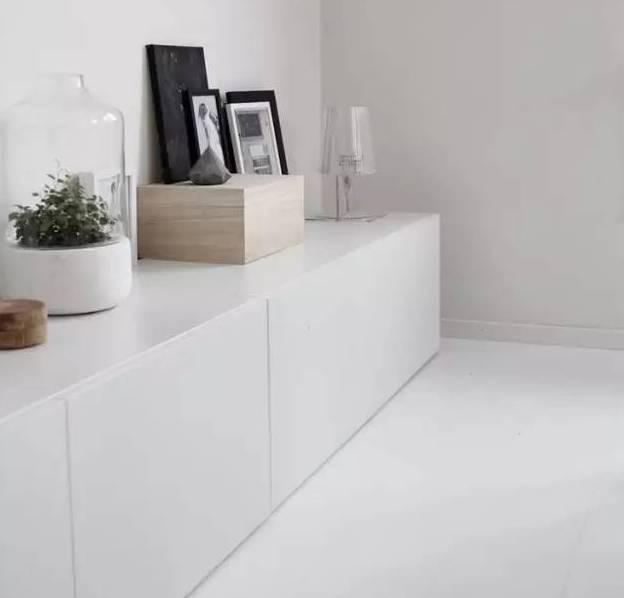镜子

▽

镜子是拓宽窄小空间的神器！

而且一面镜子能用三个作用，一是拓宽空间，二是起到装饰作用，三是兼了穿衣镜的职能。

不过有些不能放镜子的地方就不要放了，老祖宗传下来的风水学还是要讲究一些的，房君不是迷信，就是…… 哎，还是讲究一点好的嘛。这家啊，大又大的住法，小有小的住法。

但是空间扩容，是同样的重要。

图片来源于网络

文字好房子编辑整理

著作权归作者所有，转载请注明出处

－end-

`声明：本文由入驻焦点开放平台的作者撰写，除焦点官方账号外，观点仅代表作者本人，不代表焦点立场错误信息举报电话： 400-099-0099，邮箱：jubao@vip.sohu.com，或点此进行意见反馈，或点此进行举报投诉。`A B C D E F G H J K L M N P Q R S T W X Y Z
A - B - C - D - E
• A
• 鞍山
• 安庆
• 安阳
• 安顺
• 安康
• 澳门
• B
• 北京
• 保定
• 包头
• 巴彦淖尔
• 本溪
• 蚌埠
• 亳州
• 滨州
• 北海
• 百色
• 巴中
• 毕节
• 保山
• 宝鸡
• 白银
• 巴州
• C
• 承德
• 沧州
• 长治
• 赤峰
• 朝阳
• 长春
• 常州
• 滁州
• 池州
• 长沙
• 常德
• 郴州
• 潮州
• 崇左
• 重庆
• 成都
• 楚雄
• 昌都
• 慈溪
• 常熟
• D
• 大同
• 大连
• 丹东
• 大庆
• 东营
• 德州
• 东莞
• 德阳
• 达州
• 大理
• 德宏
• 定西
• 儋州
• 东平
• E
• 鄂尔多斯
• 鄂州
• 恩施
F - G - H - I - J
• F
• 抚顺
• 阜新
• 阜阳
• 福州
• 抚州
• 佛山
• 防城港
• G
• 赣州
• 广州
• 桂林
• 贵港
• 广元
• 广安
• 贵阳
• 固原
• H
• 邯郸
• 衡水
• 呼和浩特
• 呼伦贝尔
• 葫芦岛
• 哈尔滨
• 黑河
• 淮安
• 杭州
• 湖州
• 合肥
• 淮南
• 淮北
• 黄山
• 菏泽
• 鹤壁
• 黄石
• 黄冈
• 衡阳
• 怀化
• 惠州
• 河源
• 贺州
• 河池
• 海口
• 红河
• 汉中
• 海东
• I
• J
• 晋中
• 锦州
• 吉林
• 鸡西
• 佳木斯
• 嘉兴
• 金华
• 景德镇
• 九江
• 吉安
• 济南
• 济宁
• 焦作
• 荆门
• 荆州
• 江门
• 揭阳
• 金昌
• 酒泉
• 嘉峪关
K - L - M - N - P
• K
• 开封
• 昆明
• 昆山
• L
• 廊坊
• 临汾
• 辽阳
• 连云港
• 丽水
• 六安
• 龙岩
• 莱芜
• 临沂
• 聊城
• 洛阳
• 漯河
• 娄底
• 柳州
• 来宾
• 泸州
• 乐山
• 六盘水
• 丽江
• 临沧
• 拉萨
• 林芝
• 兰州
• 陇南
• M
• 牡丹江
• 马鞍山
• 茂名
• 梅州
• 绵阳
• 眉山
• N
• 南京
• 南通
• 宁波
• 南平
• 宁德
• 南昌
• 南阳
• 南宁
• 内江
• 南充
• P
• 盘锦
• 莆田
• 平顶山
• 濮阳
• 攀枝花
• 普洱
• 平凉
Q - R - S - T - W
• Q
• 秦皇岛
• 齐齐哈尔
• 衢州
• 泉州
• 青岛
• 清远
• 钦州
• 黔南
• 曲靖
• 庆阳
• R
• 日照
• 日喀则
• S
• 石家庄
• 沈阳
• 双鸭山
• 绥化
• 上海
• 苏州
• 宿迁
• 绍兴
• 宿州
• 三明
• 上饶
• 三门峡
• 商丘
• 十堰
• 随州
• 邵阳
• 韶关
• 深圳
• 汕头
• 汕尾
• 三亚
• 三沙
• 遂宁
• 山南
• 商洛
• 石嘴山
• T
• 天津
• 唐山
• 太原
• 通辽
• 铁岭
• 泰州
• 台州
• 铜陵
• 泰安
• 铜仁
• 铜川
• 天水
• 天门
• W
• 乌海
• 乌兰察布
• 无锡
• 温州
• 芜湖
• 潍坊
• 威海
• 武汉
• 梧州
• 渭南
• 武威
• 吴忠
• 乌鲁木齐
X - Y - Z
• X
• 邢台
• 徐州
• 宣城
• 厦门
• 新乡
• 许昌
• 信阳
• 襄阳
• 孝感
• 咸宁
• 湘潭
• 湘西
• 西双版纳
• 西安
• 咸阳
• 西宁
• 仙桃
• 西昌
• Y
• 运城
• 营口
• 盐城
• 扬州
• 鹰潭
• 宜春
• 烟台
• 宜昌
• 岳阳
• 益阳
• 永州
• 阳江
• 云浮
• 玉林
• 宜宾
• 雅安
• 玉溪
• 延安
• 榆林
• 银川
• Z
• 张家口
• 镇江
• 舟山
• 漳州
• 淄博
• 枣庄
• 郑州
• 周口
• 驻马店
• 株洲
• 张家界
• 珠海
• 湛江
• 肇庆
• 中山
• 自贡
• 资阳
• 遵义
• 昭通
• 张掖
• 中卫

1室1厅1厨1卫1阳台

1
2
3
4
5

0
1
2

1

1

0
1
2
3报名成功，资料已提交审核A B C D E F G H J K L M N P Q R S T W X Y Z
A - B - C - D - E
• A
• 鞍山
• 安庆
• 安阳
• 安顺
• 安康
• 澳门
• B
• 北京
• 保定
• 包头
• 巴彦淖尔
• 本溪
• 蚌埠
• 亳州
• 滨州
• 北海
• 百色
• 巴中
• 毕节
• 保山
• 宝鸡
• 白银
• 巴州
• C
• 承德
• 沧州
• 长治
• 赤峰
• 朝阳
• 长春
• 常州
• 滁州
• 池州
• 长沙
• 常德
• 郴州
• 潮州
• 崇左
• 重庆
• 成都
• 楚雄
• 昌都
• 慈溪
• 常熟
• D
• 大同
• 大连
• 丹东
• 大庆
• 东营
• 德州
• 东莞
• 德阳
• 达州
• 大理
• 德宏
• 定西
• 儋州
• 东平
• E
• 鄂尔多斯
• 鄂州
• 恩施
F - G - H - I - J
• F
• 抚顺
• 阜新
• 阜阳
• 福州
• 抚州
• 佛山
• 防城港
• G
• 赣州
• 广州
• 桂林
• 贵港
• 广元
• 广安
• 贵阳
• 固原
• H
• 邯郸
• 衡水
• 呼和浩特
• 呼伦贝尔
• 葫芦岛
• 哈尔滨
• 黑河
• 淮安
• 杭州
• 湖州
• 合肥
• 淮南
• 淮北
• 黄山
• 菏泽
• 鹤壁
• 黄石
• 黄冈
• 衡阳
• 怀化
• 惠州
• 河源
• 贺州
• 河池
• 海口
• 红河
• 汉中
• 海东
• I
• J
• 晋中
• 锦州
• 吉林
• 鸡西
• 佳木斯
• 嘉兴
• 金华
• 景德镇
• 九江
• 吉安
• 济南
• 济宁
• 焦作
• 荆门
• 荆州
• 江门
• 揭阳
• 金昌
• 酒泉
• 嘉峪关
K - L - M - N - P
• K
• 开封
• 昆明
• 昆山
• L
• 廊坊
• 临汾
• 辽阳
• 连云港
• 丽水
• 六安
• 龙岩
• 莱芜
• 临沂
• 聊城
• 洛阳
• 漯河
• 娄底
• 柳州
• 来宾
• 泸州
• 乐山
• 六盘水
• 丽江
• 临沧
• 拉萨
• 林芝
• 兰州
• 陇南
• M
• 牡丹江
• 马鞍山
• 茂名
• 梅州
• 绵阳
• 眉山
• N
• 南京
• 南通
• 宁波
• 南平
• 宁德
• 南昌
• 南阳
• 南宁
• 内江
• 南充
• P
• 盘锦
• 莆田
• 平顶山
• 濮阳
• 攀枝花
• 普洱
• 平凉
Q - R - S - T - W
• Q
• 秦皇岛
• 齐齐哈尔
• 衢州
• 泉州
• 青岛
• 清远
• 钦州
• 黔南
• 曲靖
• 庆阳
• R
• 日照
• 日喀则
• S
• 石家庄
• 沈阳
• 双鸭山
• 绥化
• 上海
• 苏州
• 宿迁
• 绍兴
• 宿州
• 三明
• 上饶
• 三门峡
• 商丘
• 十堰
• 随州
• 邵阳
• 韶关
• 深圳
• 汕头
• 汕尾
• 三亚
• 三沙
• 遂宁
• 山南
• 商洛
• 石嘴山
• T
• 天津
• 唐山
• 太原
• 通辽
• 铁岭
• 泰州
• 台州
• 铜陵
• 泰安
• 铜仁
• 铜川
• 天水
• 天门
• W
• 乌海
• 乌兰察布
• 无锡
• 温州
• 芜湖
• 潍坊
• 威海
• 武汉
• 梧州
• 渭南
• 武威
• 吴忠
• 乌鲁木齐
X - Y - Z
• X
• 邢台
• 徐州
• 宣城
• 厦门
• 新乡
• 许昌
• 信阳
• 襄阳
• 孝感
• 咸宁
• 湘潭
• 湘西
• 西双版纳
• 西安
• 咸阳
• 西宁
• 仙桃
• 西昌
• Y
• 运城
• 营口
• 盐城
• 扬州
• 鹰潭
• 宜春
• 烟台
• 宜昌
• 岳阳
• 益阳
• 永州
• 阳江
• 云浮
• 玉林
• 宜宾
• 雅安
• 玉溪
• 延安
• 榆林
• 银川
• Z
• 张家口
• 镇江
• 舟山
• 漳州
• 淄博
• 枣庄
• 郑州
• 周口
• 驻马店
• 株洲
• 张家界
• 珠海
• 湛江
• 肇庆
• 中山
• 自贡
• 资阳
• 遵义
• 昭通
• 张掖
• 中卫• 手机• 分享
• 设计
免费设计
• 计算器
装修计算器
• 入驻
合作入驻
• 联系
联系我们
• 置顶
返回顶部